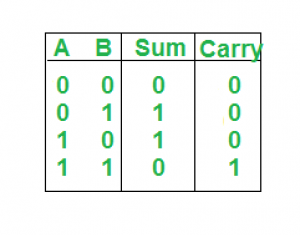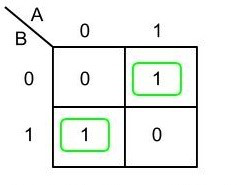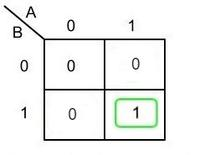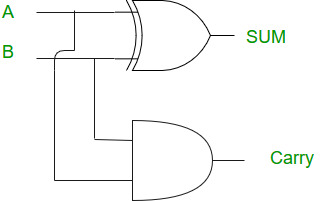# Half Adder in Digital Logic

• Difficulty Level : Easy
• Last Updated : 09 Jun, 2022

Half adder is the simplest of all adder circuits. Half adder is a combinational arithmetic circuit that adds two numbers and produces a sum bit (s) and carry bit (c) both as output. The addition of 2 bits is done using a combination circuit called a Half adder. The input variables are augend and addend bits and output variables are sum & carry bits. A and B are the two input bits.

let us consider two input bits A and B, then sum bit (s) is the X-OR of A and B. it is evident from the function of a half adder that it requires one X-OR gate and one AND gate for its construction.

Truth Table:Here we perform two operations Sum and Carry, thus we need two K-maps one for each to derive the expression.

### Logical Expression:

For Sum:Sum = A XOR B

For Carry:Carry = A AND B

### Implementation:Note: Half adder has only two inputs and there is no provision to add a carry coming from the lower order bits when multi addition is performed.

My Personal Notes arrow_drop_up
Recommended Articles
Page :